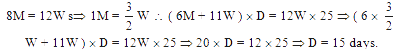# Sample Aptitude Questions of Nucleus-Software

1. There are 3 vacancies in a firm and 15 applicants. Find the total number of ways of filling these vacancies
1. 3375
2. 2730
3. 560
4. 600
5.  None of these
Reqd. number of ways = 15 × 14 × 13 = 2730
2. The number of ways of selecting four numbers from 1 to 30 so as to exclude every selection of four consecutive numbers is
1.  27378
2.  29465
3.  26368
4. 11448
5. None of these
.Reqd. number of ways = 30C4 – 27= 27378.  Here 27 are the number of ways in which we can have 4 consecutive numbers
1. A train does a journey without stopping in 8 hours. If it had traveled 5 km an hour faster, it would have done the journey in 6 hours 40 min. What is its slower speed?
1. 35 km/hr
2. 25 km/hr
3.  40 km/hr
4. 20 km/hr
5. 30 km/hr2. A person sets to cover a distance of 12 km in 45 minutes. If he covers 3/4 of the distance in 2/3 of time, then what is the speed in the remaining time?
1. 16 km/hr
2.  8 km/hr
3. 12 km/hr
4. 55 km/hr
5. 10 km/hr3. If 8 men or 12 women can do a piece of work in 25days, in how many days, can the work be done by 6 men and 11 women working together?
1. 12 days
2. 15 days
3.  9 days
4. 18 days
5. 10 days4. Some men promised to do a job in 18 days, but 6 of them became absent and remaining men did the job in 20 days. What is the original number of men?
1. 50 men
2.  60 men
3.  65 men
4. 70 men
5. 55 men
Let the number of men originally = M. According to the given condition M * 18 = ( M – 6) * 20 =>M = 60
5. The product of the digits of a two-digit number is one-third of that number. If we add 18 to the required number we get a number consisting of the same digits written in reverse order. Find the number
1. 42
2.  24
3. 72
4.  27
5. None of these
Go by options. 2nd option is correct because 24 = 3 *2 *4. Number is thrice the product of its digits.
Also 24 + 18 = 42. Hence digits are reversed.
6. Bill, Simon, and John are brothers, given Simon is the eldest. Bill is as many years younger than one brother as he is older than the other. Simon is 7 years younger than twice the age of John. John is 5 years older than half the age of Bill. What is the sum of the ages of Bill, Simon and John?
1. 12
2. 24
3.  48
4. 42
5.  Can’t say
S - B = B - J; J = B/2 + 5;
S = 2J - 7; S = B + 10 - 7 = B-3;
J= B/2 + 5 ; 2S =B + 10;
B/2 + 5 + B + 3 = 2B;
B/2 = 8 ; B = 16 ; S = 19 ; J = 13;
So B - S - J =16 - 19 - 13 = 48;
7. Cost price of each of the articles A and B is Rs. 'X'. Article A was sold at a profit of 10% and article B was sold at a profit of 30%. If the overall profit earned after selling both the articles is Rs. 136/-, what is the value of 'X'?
1. 2
2. 16
3. 44
4. 87